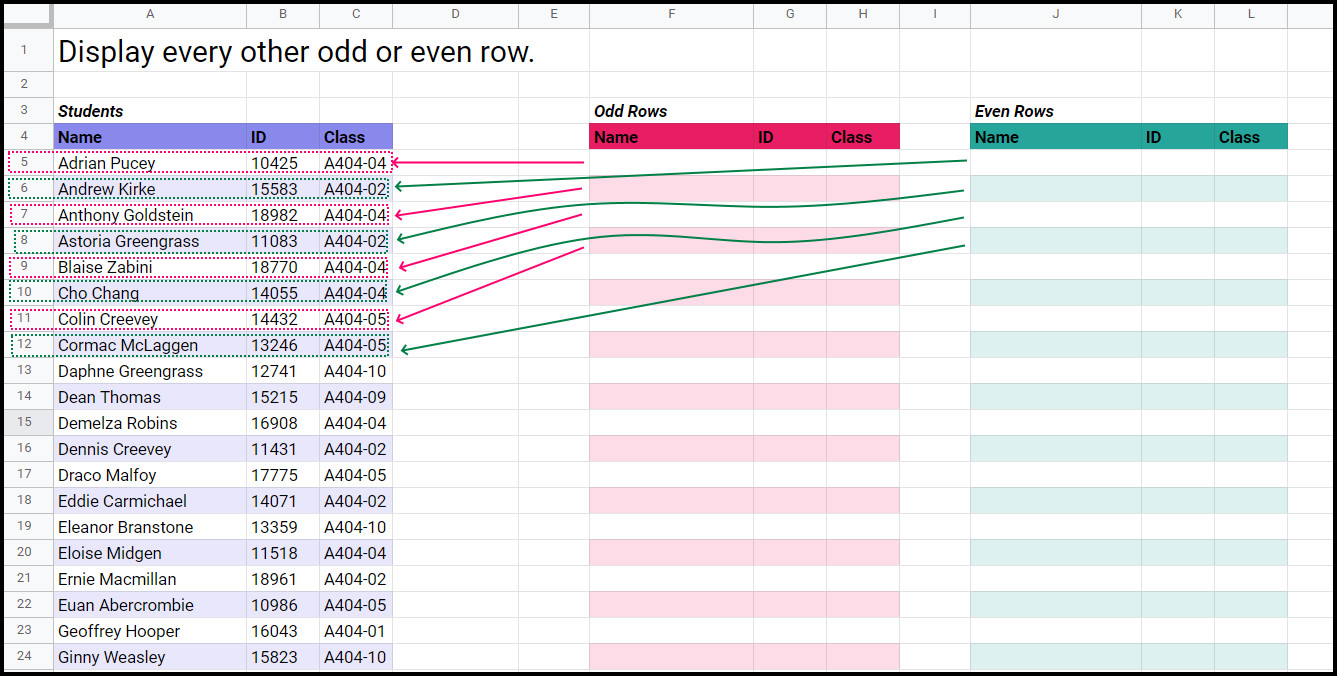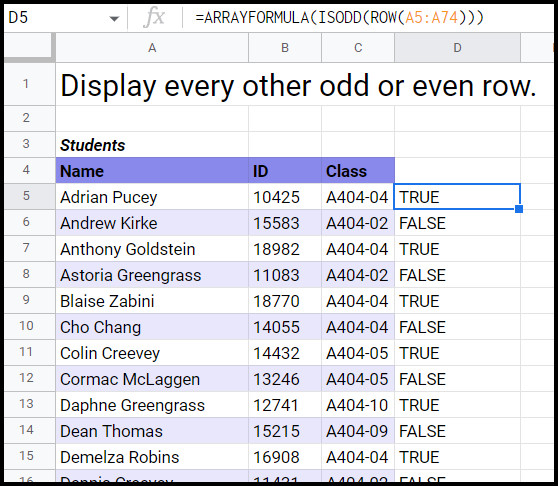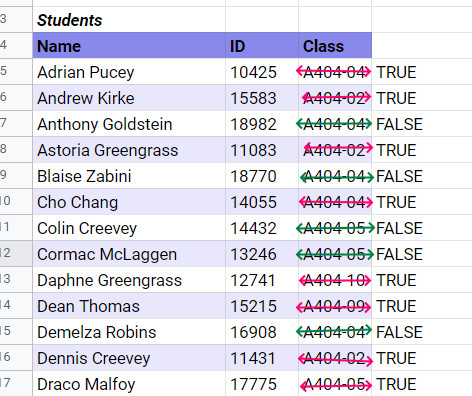# Displaying Odd and Even Row Data in Google SheetsHave you ever wanted to split a list of Google Sheet rows in two by odd or even? Or had a time when you wanted to display all items in a range by odd or even ids in two separate lists?

You don’t have to do this manually, we can work smart and create a formula for each of these scenarios to easily separate your data.

In this tutorial, we will cover three scenarios:

1. Separate a range of data by odd and even rows.
2. Separate a range by odd and even where a selected column has a list of numerical ids.
3. Separate a range by odd and even where a selected column contains a list of alphanumeric ids.

If you want to play along, you can get a copy of the starter sheet here:

Odd and Even | Starter Google Sheet*

*Contains Easter Eggs.

## Why would you want to split a range by odd and even?

You might find this a little odd (get it?), but there are some very good reasons why you may need to split a range of data by odd or even rows or values. Here are two:

• Dividing your data into two even (also… get it?) groups.
•  Sometimes ids are given odd or even values as a categorical identifier. For example, all staff members who have an odd id belong to a late shift.

## Display every other odd or even row

In this example, we want to evenly split our range of students. We can do this by generating a list of students in every other row where the row number is either even or odd.

Check out the sample data:In the example above, you can see that we want to put all odd the sheet rows in the ranges from `A5:A74` into columns `F:H`. Likewise, we want to add all the even rows from `A5:A74` into columns `J:L`.

Here is the formula for even and odd ranges:

• Odd (cell F5): `=``FILTER(A5:C74, ARRAYFORMULA(ISODD(ROW(A5:A74))))`
• Even (call J5): `=FILTER(A5:C74, ARRAYFORMULA(ISEVEN(ROW(A5:A74))))`

This will give us this:

### Formula Breakdown

#### FINDING THE ROW

First, we need to find out which row each student is on. We can do this with the Google Sheets ROW function. This function takes a cell reference as an argument.

So if I were to select Adrian Pucey’s cell (`A5`) as an argument then my result would be 5.

`=ROW(A5) = 5 `

#### iS THE ROW ODD OR EVEN?

We can then use the ISODD or ISEVEN functions to determine if the cell number is even or odd. These functions take a number and return either true or false. So using our `A5` example again the formula would look like this:

• `=ISODD(ROW(A5)) = ISODD(5) = true`
• `=ISEVEN(ROW(A5)) = ISEVEN(5) = false`

#### CHECKING THE ENTIRE RANGE

Now, we want to check all the rows in our selected column to see if their row number is odd or even. We can do this with the ARRAYFORMULA function. This function allows us to apply formulas over a range of data instead of a single cell. ARRAYFORMULA takes a formula as an argument.

Let’s go ahead and select the range containing all of our student names and add this to our formula:

`=ARRAYFORMULA(ISODD(ROW(A5:A74)))`

This will give us a column of all the rows as either true or false. If we were to add this formula to cell D5, it would look like this:#### CREATING A FILTER FOR ODD OR EVEN ROWS

Our last task is to create a filter to… well… filter out the odd or even row. We can do this with the Google Sheets FILTER function. FILTER takes a range to display as the first argument and then filter parameters as consecutive arguments.

Let’s take a look at our formulas again.

• `=FILTER(range_to_display, filter_parameter)`
• Odd: `=``FILTER(A5:C74, ARRAYFORMULA(ISODD(ROW(A5:A74))))`
• Even: `=FILTER(A5:C74, ARRAYFORMULA(ISEVEN(ROW(A5:A74))))`

Here we are saying, only display rows in range `A5:C74` where row numbers are either even or odd, depending on what we want to show.

### The Video

Scheduled release 02 June 2022

## Display All Rows With Even or Odd Student IDs

In this example, we want to display all students who have an even ID in column F and all students with an even student ID in column J.

Check out the formula:

• Odd (cell F5): `=``FILTER(A5:C74, ARRAYFORMULA(ISODD(B5:B74)))`
• Even (call J5): `=FILTER(A5:C74, ARRAYFORMULA(ISEVEN(B5:B74)))`

### Formula Breakdown

We will be running through a similar process as the previous example here, so I won’t go into as much detail.

This time around, we want to create an array that checks the value of the student ID in column B and returns true or false if it is even or odd.

This is what our ARRAYFORMULA would look like for all odd student IDs on its own:

• `=ARRAYFORMULA(ISODD(B5:B74))`## Display All Rows With Even or Odd Classes

In this final example, we want to display all the rows containing students with either odd or even classes. We will probably also want to sort our students by their classes too. Our end result should look a little like this:Now we can’t use the same formula as before ISODD and ISEVEN only take numbers as arguments, but our class ids contain letters and a hyphen. We need a workaround for this. Check out the code:

• Odd (cell F5): `=SORT(FILTER(A5:C74, ARRAYFORMULA(ISODD(RIGHT(C5:C74)))),3, TRUE)`
• Even (call J5): `=SORT(FILTER(A5:C74, ARRAYFORMULA(ISEVEN(RIGHT(C5:C74)))),3, TRUE)`

### Formula Breakdown

#### Get the digit at the end

We can see from our class id data, that each class ends in a number (e.g. A404-04, A404-05). We can extract that last number with the RIGHT function. RIGHT extracts a portion of a string at a set length from the right of the text. The function takes a cell reference as an argument and you can also add a length of characters to collect as a second argument, but we won’t be doing that here.

If we were to use RIGHT on cell C5 on its own, it would look like this:

`=RIGHT(C5) = RIGHT("A404-04") = 4`

We can then check if that number is odd or even.

#### Sorting the data by Class ID

We can sort our data by class ID by using the Google Sheets SORT function. SORT takes a range as its first argument and then in argument pairs, it takes a column to sort and whether that column should be sorted in ascending or descending order.

`=SORT(range to sort, column number, ascending/descending, col num, asc/desc, ... , ...)`

In our example, we want to sort by the third column, the class id, and sort that column from the smallest to the highest class (Ascending order)

• `=SORT(filtered range,3, TRUE)`

### The Video

Scheduled release 3 June 2022

## Conclusion

That’s three ways to filter odd and even rows and values in a range in Google Sheets. I’d love to hear how you used these examples in your own projects in the comments below. Your ideas and approaches will inspire others.

If you have found the tutorial helpful, why not shout me a coffee ☕? I'd really appreciate it.

Did you enjoy the tutorial? Want to upskill and get a solid step-by-step course to become a pro at Google Sheets? Check out my course, Google Sheets: Learn the Essentials with Three Detailed Projects. Sign up today.